## Reverse engineering the Smaz compression library

time to read 9 min | 1737 words

Smaz is a small library for compressing small strings. It is strange to talk about reverse engineering such a project, because Smaz is open source and is available on GitHub. Nevertheless the code on GitHub is actually only part of the story. It is dense,  and it was generated by a script that isn't included. That means that when we read the code, we are missing some key components, in particular, you are looking at an end result, without knowing how you got there.

Compression algorithms take advantage of repetitions in text to reduce the overall size. State of the art algorithms typically use Lempel-Ziv and Huffman coding to compress the data. But because they are typically making no assumptions about the data, and they require a bit of length before they can gather enough state to truly gather some steam and start reducing the output length.

That means that for small strings (up to a hundred bytes or so), there is no real benefit in standard compression algorithms, and often you'll see an increase in the space taken. Smaz is meant to solve that for most common cases. It does so by using a prepared dictionary of common terms that can be easily compressed.

The first thing that you'll encounter when you read the Smaz code is this:This goes on for about 50 lines, and at first glance, it looks utterly incomprehensible. We'll ignore this for now, and read further. The next item of interest is: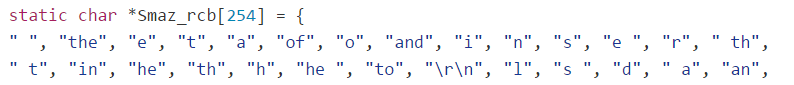The cb postfix stands for code book, and rcb stands for reverse code book.

It took me a while to figure it out, but the idea in the code book entries is that this is actually a hash table. Each string in the codebook is actually multiple entries, composed of a single byte length, the term text, and then the index in the codebook. There are possibly multiple entries in each value in the array, and we use the length of each entry to index into them.

Let us see how this works:

```int smaz_compress(char *in, int inlen, char *out, int outlen) {
unsigned int h1,h2,h3=0;
int verblen = 0, _outlen = outlen;
char verb, *_out = out;

while(inlen) {
int j = 7, needed;
char *flush = NULL;
char *slot;

h1 = h2 = in<<3;
if (inlen > 1) h2 += in;
if (inlen > 2) h3 = h2^in;
if (j > inlen) j = inlen;
```

This code just setup a temporary buffer, and hash the current input term's bytes. Then we start getting interesting.

```        /* Try to lookup substrings into the hash table, starting from the
* longer to the shorter substrings */
for (; j > 0; j--) {
switch(j) {
case 1: slot = Smaz_cb[h1%241]; break;
case 2: slot = Smaz_cb[h2%241]; break;
default: slot = Smaz_cb[h3%241]; break;
}
while(slot) {
if (slot == j && memcmp(slot+1,in,j) == 0) {
/* Match found in the hash table,
```

The value j is the current size we are checking, and we are using the hash value to get a particular slot in the hash table. Note that only the first 3 bytes are actually hashed. After the appropriate slot is found, we check whatever the term length is a match, and if so, whatever the actual strings match. If they don't, we run the following code:

```slot += slot+2;
```

this was a bit confusing at first, but what is basically going on here is that we move to the next entry in this slot. This is pretty neat, since it covers both empty slots (defined in the code as "") and multi value slots and take advantage on the fact that C strings end with \0 without having to specify it explicitly.

So what happens if we have a match?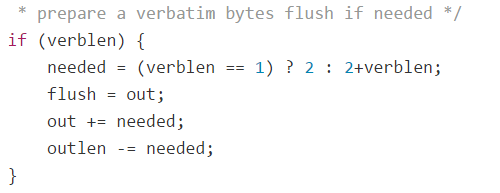In this case, we check if there are any verbatim bytes (bytes that we haven't been able to compress that are stored in a buffer.). If there are such bytes, we do the following:

• Compute the space needed for the verbatim value.
• Set the next flush point in the buffer for verbatim values to the current output location.
• Move the output pointer after the location where the verbatim string will be written.
• Reduce the length of the remaining output size by the verbatim length.

Note that we aren't actually going to write anything yet. First, we need to emit the newly captured match to the code book: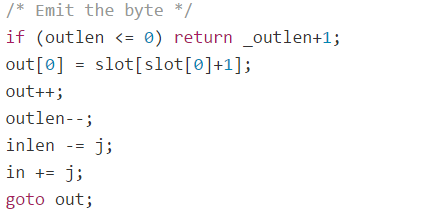This is done by first checking if there is space (if there isn't, we return an error).

Then we write a byte to the output buffer. But this is a very strange byte.

What is this "slot[slot+1]" ? Well, remember the structure that we talked about for the hash table entries? The first byte is a length, and the last byte is the index into the code book. So what we are actually doing is indexing into the entry to get the last value, which his the code book index, which we write to the output.

The rest is pretty much just book keeping information. We move the input buffer pointer according to the just discovered term, etc.If no match was found, we just add the current byte to the verbatim buffer, and move on to the next one.

Now, let us look at this out label, and what it does: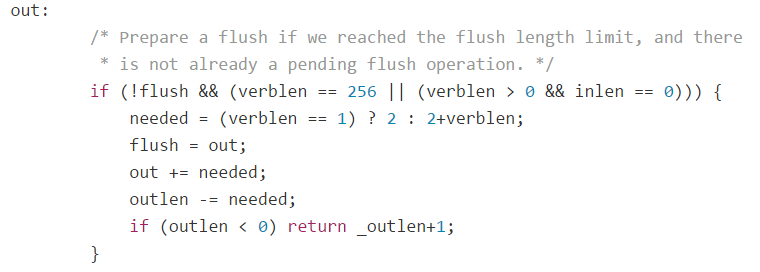Basically, it is a repeat of the earlier code when finding a match. If we have a large enough verbatim string, we need to flush it, so this takes care of it. The actual flushing is interesting: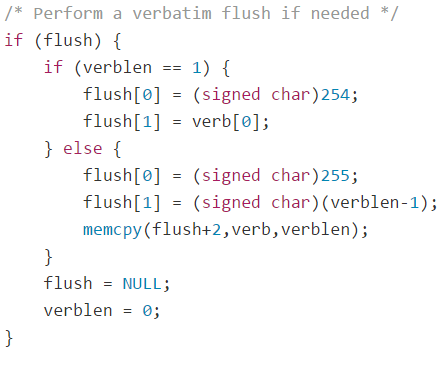If there is a single byte, we write 254 (single verbatim byte marker), then we write the byte. If there is more than a byte, we write 255 (variable length size), the length, then the verbatim string.

It is interesting to note that this can be written after the output byte has been written. It took me a while to understand this. Nothing wrong with it, but I like sequential writes much better.

The decompression is very simple. Read a byte from the compressed input, if it is  254, then the next byte should be copied verbatim to the output. If the byte is 255, then read the next byte, which is the length, and copy the next length bytes to the output. Finally, if the byte isn't 255 or 254, it is an index into the code book table, and the value should be taken from there.

I wrote a managed implementation of this, which allows me to play much more easily with the codebook definition. This was because there are a bunch of terms in the Smaz codebook that aren't really relevant for what we need. In particular, it looks like it was trained to find the most common values from a set of HTML documents, it has entries for "<div>", "><", etc. Instead of going with the 254 values that can be compressed, I pruned the list a bit, and ended up with just 245 items.

I was then able to change the compression format to be:

• If it is a value under 245, use the term from the codebook.
• If it is a value higher than 245, it is a verbatim value whose actual length need to be figured out by subtracting 245 from it.

This allows me to save a byte if we have more than two verbatim bytes.

Smaz is a really simple and clean library, but it isn't doing something very complex. It is a static shared dictionary approach, without the use of more advanced approaches. I previously written about a more complex compression systems for small strings, such as FemtoZip. You can find the previous post here (there was a whole series of them).

In my next post, I'll try to compare the two options.